# Orbital Mechanics

At the outset, I want to make one thing crystal clear to the reader: I am not a scientist, mathematician, or technologist. I do not purport to be any type of subject-matter expert. I am simply a writer who has conducted a little research. As a result, the following explanation of satellite orbits will be very basic and simple. The discussion will focus on circular artificial satellite orbits. In keeping it simple, the long-form representation of numbers will be used rather than the short-form, scientific-notation format. Substantial portions of the materials covered on this page were gleaned from websites such as braeunig.us. The purpose of this discussion is to provide the reader with something of a behind-the-scenes look at the mechanics of artificial satellite orbits.

The shape of Earth closely approximates the shape of a round, 3-dimensional sphere (for example, a marble, grapefruit, or soccer ball). For illustrative purposes here, it will be simpler to depict Earth's shape as that of a flat, two-dimensional circle (for example, a coin, music CD, or round serving plate).

To keep the explanation of artificial satellite orbits as simple as possible, this page will focus on circular artificial satellite orbits. As the next graphic illustrates, the two-dimensional circle exhibits multiple properties. The graphic also lists some formulas commonly used to work with circles. This discussion will be limited to the circumference, diameter, radius, and pi properties of circles.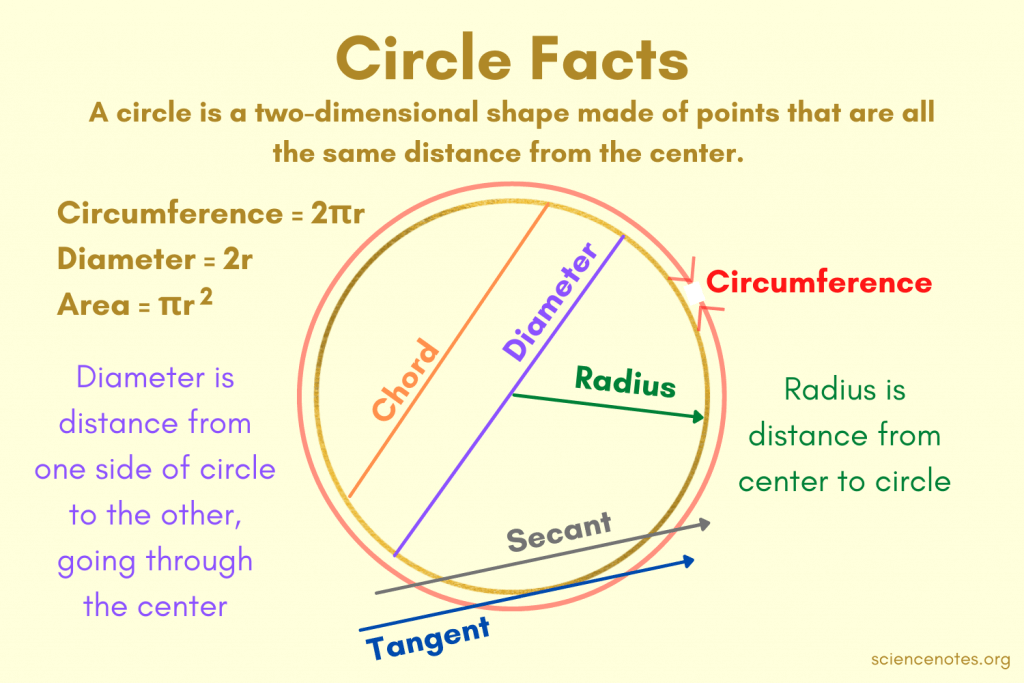As for pi (π), the properties of circles have been studied since ancient time. According to the piday.org website, it was the great Greek mathematician, Archimedes (about 250 B.C.E.), who deduced that the value of pi approximates 22 ÷ 7. Pi's value later would be refined to approximate 3.141592653589793…. Pi has proven to be an invaluable calculation tool when working with circles, in general, and when working with the Earth's circular shape, in particular. The most commonly used formula to compute the value of pi is π = Circumference ÷ Diameter. When working with circles, regardless of the values for the variables C and D in the pi formula, the solution is always 3.14….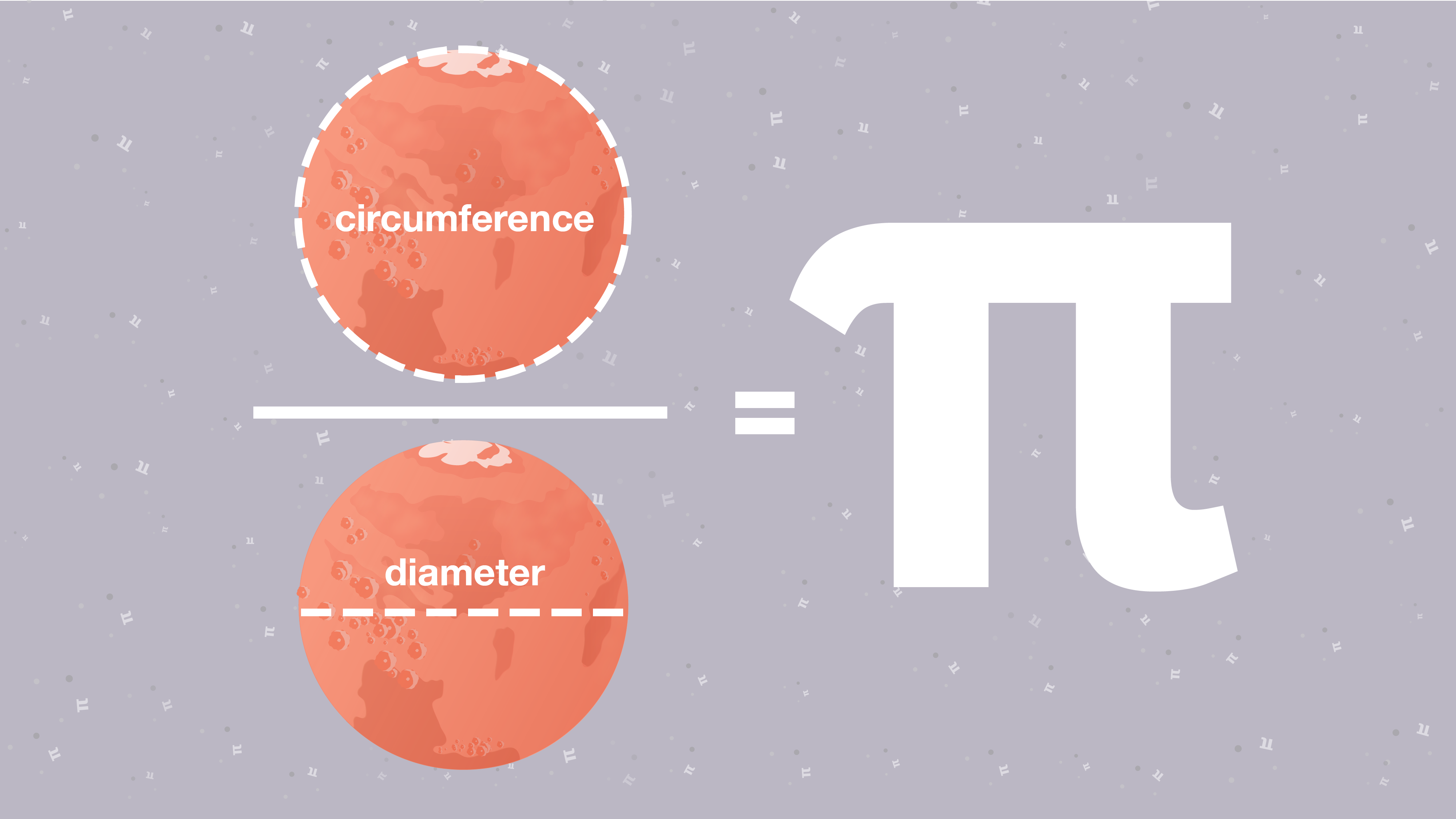In addition to the properties of circles, in computing artificial satellite orbits, it is useful to start with pre-computed values that have been verified by scientists and mathematicians. These pre-computed values supply the values for the variables used in an equation. It then becomes a matter of simply plugging or substituting the values for the applicable variables and, voilà, the solution is obtained to the formula. Some of these pre-computed values include the following:

• Earth's equatorial circumference: 40075.017 (km)
• Earth's mean equatorial radius r: 6378.1 (km)
• Earth's diameter: 12756 (km)
• Earth's mass M: 5.9736 (10²⁴kg)
• Earth's equatorial gravity: 9.80 (m/s²)
• Earth's escape velocity: 11.19 (km/s⁻¹)
• Earth's rotation period T: 23.9 (hours) / 86400 seconds
• Newtonian universal gravitational constant G: 6.67428 × 10⁻¹¹ kg ⁻¹ m³ s⁻²
• Earth's gravitational constant GM: 3.986005 × 10¹⁴ (m³/s²)

• Take, the circle's circumference formula, for instance. Given the known values for pi and given the known value for the Earth's radius, it becomes simply a matter of plugging those values into the circumference formula to determine Earth's equatorial circumference as shown in the next graphic.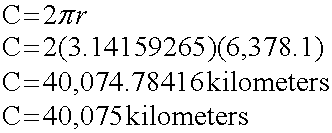The artificial satellite story can be traced back to the great scientist and mathematician named Sir Isaac Newton (born 1643 and died 1727 at age 84). The next graphic outlines Sir Issac Newton's Universal Law of Gravitation. In essence, the law states that the force (gravity) attracting two objects such as the Earth and the Moon or the Earth and an artificial satellite is equal to the gravitational constant (G) multiplied by the masses of the two objects (m1 and m2) and divided by the square of the distance (r²) between them. This discovery by Sir Issac Newton has proven to be an absolute treasure trove for scientists and mathematicians.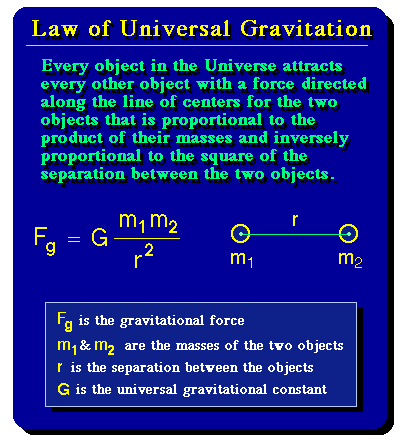As depicted in the next graphic, in conjunction with a circle (such as Earth), the key to computing satellite orbits resides with computing the values for the variables a and v. Fortunately, mathematicians have determined the formulas used to obtain values for these two variables.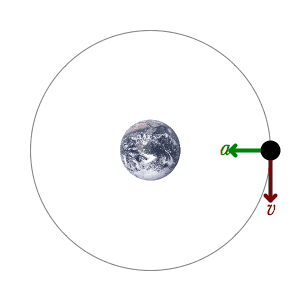The first step in understanding how artificial satellites orbit would be to determine the acceleration of gravity at Earth's surface. To know Earth's acceleration of gravity is to know the minimum speed (hence, force) needed to overcome this particular acceleration of gravity. Fortunately, mathematicians already have done the hard work in making this determination. Based on Sir Isaac Newton's work, mathematicians have derived formulas used to compute, among other things, the acceleration of gravity on Earth. Gravity's acceleration value at or near the Earth's surface (9.80665 m/s²) can be determined by using the following derived formula: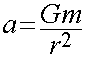Where,

• a = acceleration due to the force of gravity (unknown value)
• G = Gravitational constant (6.67428 × 10⁻¹¹ m³ kg⁻¹ s⁻²)
• m = mass of Earth (5.9736 × 10²⁴ kg)
• r = radius of Earth (6,378.1 kilometers or 6,378,100 meters)After plugging the values for the variables G, m, and r, into the acceleration formula, the solution becomes 9.80665 meters per second, per second for gravity's acceleration rate at or near the Earth's surface.

The second step in understanding how artificial satellites orbit would be to determine Earth's escape velocity. Escape velocity means to overcome or leave the gravitational influence of a heavenly body such as a planet like Earth. When a spacecraft or satellite leaves planet Earth and travels into outer space, say, to the Moon, Mars, or Pluto, then it is said to have escaped the gravitational influence of Earth.

By knowing the value for Earth's escape velocity, then a maximum speed can be computed for launching a satellite into outer space. If, say, an artificial satellite is accelerated straight into the sky too fast at launch (that is, more than 11 kilometers per second), then it would escape the hold of Earth's gravity and would continue to travel beyond planet Earth and out into the wider Solar System. If an artificial satellite is accelerated too slowly at launch, then it would fall back down to Earth under the influence of Earth's gravity. Again, mathematicians have derived formulas to compute the Earth's escape velocity. One simple derived formula used to compute Earth's escape velocity is the following one:Where,

• v = escape velocity (unknown value)
• g = gravity's acceleration at Earth's surface (9.80665 m/s² or meters per second squared)
• r = radius of Earth (6,378.1 kilometers or 6,378,100 meters)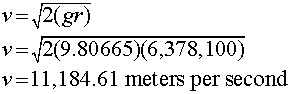After plugging the values for the escape-velocity variables g and r into the escape-velocity formula, the solution for the Earth's escape velocity becomes 11,184.61 meters per second. Using the metric system, the 11,184.61 meters per second translate into an escape velocity from Earth of 40,264.6101 kilometers per hour (that is, 11,184.61 meters × 60 seconds = 671,076.6 meters in a minute; and 671,076.6 meters × 60 minutes = 40,264,596 meters per hour; and 40,264,596 meters ÷ 1,000 meters in a kilometer = 40,264.6 kilometers per hour). Using the USA's measurement system, the 11,184.61 meters per second (or 36,694.9147 feet per second) translate into an escape velocity from Earth of 6.94979689 miles per second or 25,019.2688 miles per hour (that is, 36,694.9147 feet × 60 seconds = 2,201,694.882 feet in a minute; and 2,201,694.882 feet × 60 minutes = 132,101,692.92 feet per hour, and 132,101,692.92 feet ÷ 5,280 feet in a mile = 25,019.26 miles per hour).

The next graphic shows another derived formula that can be used to compute the Earth's escape velocity.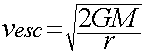The third and final step in understanding how artificial satellites orbit would be to determine Earth's orbit velocity. When the acceleration (a), velocity (v) dynamic is in harmony, and with all other things remaining equal such as (a) an absence of atmospheric drag, (b) no change in propulsion affecting the artificial satellite's speed and direction, and (c) no unforeseen events like satellite malfunctions or collisions, then a state of circular orbit is achieved by the artificial satellite. At orbital velocity, once an artificial satellite is released by the spacecraft at a given height and speed, in theory, it could orbit Earth forever much like the Moon is perpetually orbiting Earth or much like the planets are perpetually orbiting the Sun. As derived by mathematicians, the next graphic gives the formula used to attain circular orbits. The next graphic assumes the artificial satellite will be placed into orbit at an altitude of 200 kilometers (or 200,000 meters).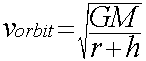Where,

• v = velocity at orbit (unknown value)
• GM = Earth's gravitational constant (3.986005 × 10¹⁴)
• r = radius of Earth (6,378.1 kilometers or 6,378,140 meters)
• h = height of the artificial satellite (200 kilometers or 200,000 meters)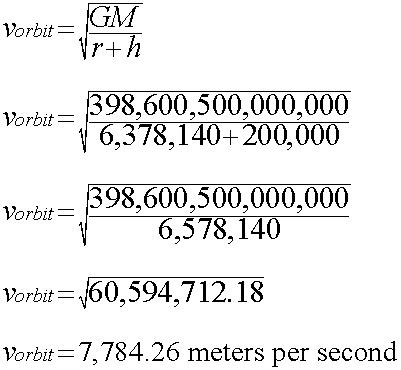The above orbital-speed example indicates that, to be placed at an altitude of 200 kilometers, the artificial satellite will require a speed of 7,784.26 meters per second to acquire a circular orbit above Earth. This speed translates into 7.78426 kilometers per second or 28,023.336 kilometers per hour.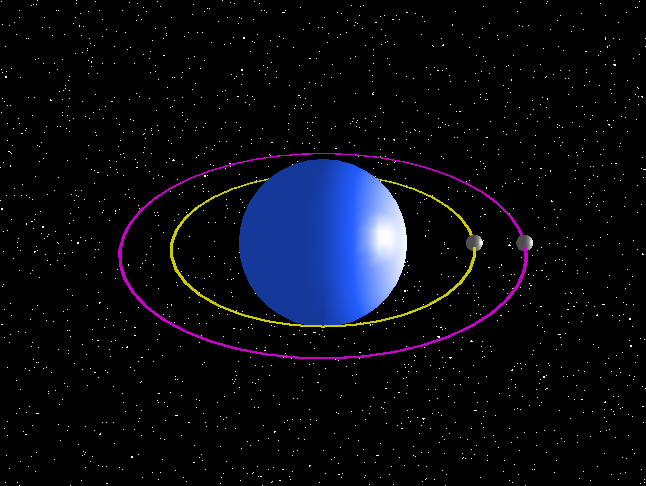When the orbital speed (v) is known, the artificial satellite's orbital period (T) around Earth can be derived by using the following formula: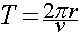Where,

• T = Time period it takes the artificial satellite to complete an orbit of Earth (unknown value)
• v = velocity or speed of the artificial satellie as meters per second (7,784.26)
• π (pi) = 3.14159265
• r = radius of Earth (6,378.1 kilometers or 6,378,140 meters) plus the height of this example artificial satellite (200 kilometers or 200,000 meters)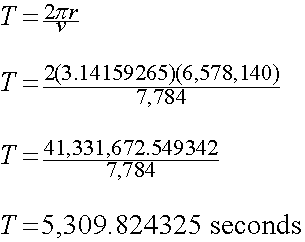The above orbital-time-period example indicates that the artificial satellite makes a complete orbit of Earth once every 5,309.82 seconds. This time period translates into once every 88.49707209 minutes or once every 1.474951202 hour.

The next graphic shows another derived formula that can be used to compute an artificial satellite's orbital period.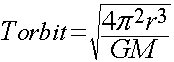The following video provides additional insights into the mathematics behind artificial satellite orbits.

Watch (Speed of a Satellite in Circular Orbit, Orbital Velocity, Period, Centripetal Force, Physics Problem)

Much like Archimedes demonstrated that 223 ÷ 71 < pi < 22 ÷ 7, it was for modern-day mathematicians to determine that, in the case of Earth's orbital mechanics, 9.8 ms < Earth orbit < 11.2 kms. Contemporary mathematicians have used Sir Isaac Newton's work to derive all kinds of calculations related to artificial satellite orbits. To be sure, many more advanced mathematical computations are involved in placing artificial satellites into orbit around Earth than the rather simplistic overview provided on this page.

Determining the mathematical key to artificial satellite orbits is one thing. Manufacturing an artificial satellite and launching it into orbit are completely different challenges altogether. Accomplishing these additional technological feats of building and launching artificial satellites is a challenge that falls within the fields of rocket science and satellite technology.